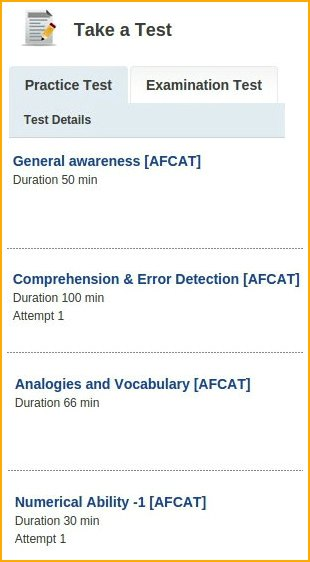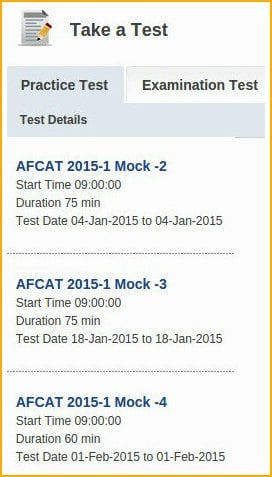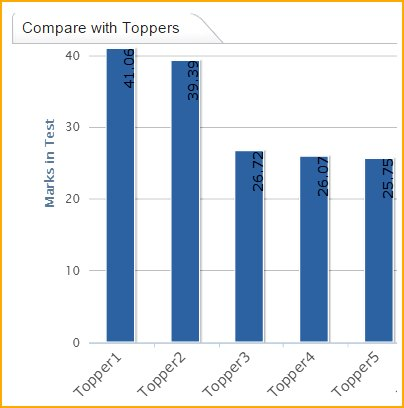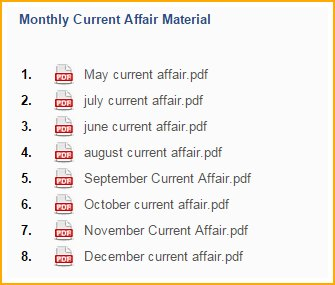# How to Prepare for AFCAT 1 2017 Exam in 15 Days

AFCAT Exam is the Air Force Common Entrance test, which is conducted by the Indian Air Force twice every year. Through this exam men and women can apply for different branches of the Indian Air Force. These branches include the Flying Branch, Technical Branch and Ground Duty Branch. By study it’s been noticed that most of the aspirants who attend AFCAT are either from college final year or working job professionals, it means for most of the AFCAT appearing candidates’ preparation for AFCAT is not in their main curriculum since they are already occupied by the busy academic schedule or job working schedule. Such aspirants preparation is often called nick-of-time raw preparation. AFCAT exam syllabus or AFCAT exam pattern are not very hard to crack as compared to other national level competitive exams, questions asked from the syllabus are very basic and can be mastered with a little bit of hard work, but as mentioned before appearing candidates don’t have any extra time schedule for the preparation of the exam as a result in AFCAT it’s been noticed that potential candidates with good intellect  are not been able to answer basic questions which further becomes very irritating for them and it’s all because they don’t have enough time to practice.

How to Prepare for AFCAT 1 2017 Exam in 15 DaysIn this article we will discuss about How to Prepare for AFCAT Exam in 15 Days. AFCAT aspirants generally start their preparation just 10-15 days before the written exam, as the time in hand is very less so everything from here a candidate should do needs to be in right direction and accurate. Below given are some pointers which may help a candidate in his/her crash course preparation:

• There are few simple AFCAT exam numerical ability maths formulas you should keep on your finger tips to solves the mathematics question of AFCAT question paper. AFCAT exam paper consists of very basic mathematics questions and these formulas will help you to clear them easily:
• Decimal Fraction: A decimal fraction is a fraction in which denominator is an integer power of ten. (The term decimals are commonly used to refer decimal fractions). Generally, a decimal fraction is expressed using decimal notation and its denominator is not mentioned explicitly.
• Conversion of a Decimal into Common Fraction: Put 1 in the denominator under the decimal point and annex with it as many zeros as is the number of digits after the decimal point. Now, remove the decimal point and reduce the fraction to its lowest terms.

Example: 0.5 = 5/10=1/2

• Some Basic Formulas:
1. (a + b)(a – b) = (a2 – b2)
2. (a + b)2 = (a2 + b2 + 2ab)
3. (a – b)2 = (a2 + b2 – 2ab)
4. (a + b + c)2 = a2 + b2 + c2 + 2(ab + bc + ca)
5. (a3 + b3) = (a + b)(a2 – ab + b2)
6. (a3 – b3) = (a – b)(a2 + ab + b2)
7. (a3 + b3 + c3 – 3abc) = (a + b + c)(a2 + b2 + c2 – ab – bc – ac)
8. When a + b + c = 0, then a3 + b3 + c3 = 3abc.
• Simplification: Rule of ‘BODMAS’ – This rule depicts the correct sequence in which the operations are to be executed, so as to find out the value of given expression. Full form of BODMAS is B – Bracket, O – of, D – Division, M – Multiplication, A – Addition and S – Subtraction. Thus, while solving or simplifying a problem, first remove all brackets, strictly in the order (), {} and ||. After removing the brackets, we will use the following operations strictly in the following order: (i) of (ii) Division (iii) Multiplication (iv) Addition (v) Subtraction.
• Average:

Average = (Sum of observations/Number of observations)

• Percentage:
1. By a certain percent, we mean that many hundredths. Thus, x percent means x hundredths, written as x%. To express x% as a fraction: We have, x% = x/100
2. To express a/b as a percent: We have, a/b = (a/b x 100)%
3. Percentage Increase/Decrease: If the price of a commodity increases by R%, then the reduction in consumption so as not to increase the expenditure is: [(R/(100+R)) x 100]%. If the price of a commodity decreases by R%, then the increase in consumption so as not to decrease the expenditure is: [(R/(100-R)) x 100]%.
4. Result on Population: Let the population of a town be P now and suppose it increases at the rate of R% per annum, then: population after n years = P (1+(R/100))n Population n years ago= P/ (1+(R/100))n
5. Result on Depreciation: Let the present value of a machine be P. Suppose it depreciates at the rate of R% per annum. Then: Value of the machine after n years = P (1-(R/100)n , Value of the machine n years ago = P/ (1-(R/100)n, If A is R% more than B, then B is less than A by [(R/(100+R)) x 100] %, If A is R% less than B, then B is more than A by [(R/(100-R)) x 100] %
• Ratio:
1. The ratio of two quantities a and b in the same units, is the fraction and we write it as a : b. In the ratio a : b, we call a as the first term or antecedent and b, the second term or consequent. Eg. The ratio 5 : 9 represents 5/9 with antecedent = 5, consequent = 9.
2. Rule: The multiplication or division of each term of a ratio by the same non-zero number does not affect the ratio. Eg. 4 : 5 = 8 : 10 = 12 : 15. Also, 4 : 6 = 2 : 3.
• Proportion: The equality of two ratios is called proportion. If a : b = c : d, we write a : b :: c : d and we say that a, b, c, d are in proportion. Here a and d are called extremes, while b and c are called mean terms. Product of means = Product of extremes. Thus, a : b :: c : d (b x c) = (a x d).
• Simple Interest:
1. Principal: The money borrowed or lent out for a certain period is called the principal or the sum.
2. Interest: Extra money paid for using other’s money is called interest.
3. Simple Interest (S.I.): If the interest on a sum borrowed for certain period is reckoned uniformly, then it is called simple interest. Let Principal = P, Rate = R% per annum (p.a.) and Time = T years. Then Simple Interest = (P x R x T)/100
• Profit and Loss:
1. Cost Price: The price, at which an article is purchased, is called its cost price, abbreviated as C.P.
2. Selling Price: The price, at which an article is sold, is called its selling prices, abbreviated as S.P.
3. Profit or Gain: If S.P. is greater than C.P., the seller is said to have a profit or gain.
4. Loss: If S.P. is less than C.P., the seller is said to have incurred a loss.
5. Gain = (S.P.) – (C.P.)
6. Loss = (C.P.) – (S.P.)
7. Loss or gain is always reckoned on C.P.
8. Gain Percentage: (Gain %) = (Gain x 100) / C.P.
9. Loss Percentage: (Loss %) = (Loss x 100) / C.P.
10. Selling Price: (S.P.) = [ ((100 + Gain %)/100) x C.P.]
11. Selling Price: (S.P.) = [ ((100 – Loss %)/100) x C.P.]
12. Cost Price: (C.P.)= [ (100/(100 + Gain %)) x S.P.]
13. Cost Price: (C.P.)= [ (100/(100 – Loss %)) x S.P.]
14. If an article is sold at a gain of say 10%, then S.P. = 110% of C.P.
15. If an article is sold at a loss of say, 20% then S.P. = 80% of C.P.
16. When a person sells two similar items, one at a gain of say x%, and the other at a loss of x%, then the seller always incurs a loss given by: Loss % = (x/10)2
17. If a trader professes to sell his goods at cost price, but uses false weights, then Gain % = [(Error/(True Wight – Error)) x 100 ]%
• Prepare with good AFCAT exam books: AFCAT exam preparation books which are available in the market are just like any other question bank, since the time in hand is less be selective when choosing topics to prepare, better to go for topics on which you have prior basic knowledge. Practice as much as questions possible, study solved examples very closely and sort out the pattern for similar type of questions.
• Use internet: Browse internet for current year and previous year current affairs read and remember as much as possible.
• Develop proper time management skill for answering maximum number of questions within the time frame, to do such search for practice test and mock test available at any online or physical coaching.
• Spatial ability is also one of the easy sections in AFCAT exam, 80-90% candidates can get more marks in spatial ability and military aptitude sections of AFCAT exam. Only thing you need is to know what kind of questions you will face in spatial ability and mental ability sections of AFCAT exam.
• History, Sports, Geography, Environment, Civics, Defence, Art, Culture, and Politics: These are the topics on which knowledge based questions are asked, instead of preparing for each topic select one third of the topics of your choice and interest and gather important facts on those topics as much as possible. Make flash cards of the information gathered and read whenever you have time in hand.
• Prepare with AFCAT previous question papers: This is considered as one of the best methods to prepare for any exam. AFCAT previous question papers would help you to understand the syllabus, pattern and types of questions you might face during AFCAT exam. Below listed are links for previous year question papers:
• Prepare with SSBCrackExams: Our AFCAT Exam + EKT preparation course:

These courses are customized to fulfil the needs of today’s AFCAT exam aspirants, AFCAT online coaching would help you to understand the concepts of AFCAT exam paper and the high end reporting tool helps you analyze your current standards by comparing with other defense aspirants who are writing AFCAT exam along with you. So, before facing the actual AFCAT exam you could look at your preparations and act accordingly. Having said that, this course will focus more on the questions which likely to come so there is no or less scope of failure. A daily practice (even before 15 days of the real exam) of SSBCrack Exams courses can do wonders for you and will surely help you to put extra marks in your bag.

SSBCrack Exams offering to AFCAT aspirants:

• AFCAT full Online Course
• AFCAT Practice Tests• AFCAT based National Level Mock Tests• High end Reports and Analysis on student performance• Expected important questions for upcoming AFCAT along with monthly GK and Current affair updatesNote: Soon we will be adding video tutorials on technical concepts to help candidates understand the concept in a more dynamic way.

To enrol in SSBcrack Exams, first a student has to register in SSBCrcak Exams website. There are two ways a student can register:

• Student can register through our website by providing – Username, Email id and Password. To Register Click Here
• Student can register with his Facebook id and password. To Register Click Here

Note:- To join online AFCAT coaching a student a have to first sign in  to portal using his/her registered login ID and password, once a student is logged in look in left hand side of the page and search for ” buy package ” and click on it. The test selection page will open, then the student have to select the respective choice of exam and click on “buy now”.#### SSBCrackExams

SSBCrackExams is a premium online portal for Indian Defence aspirants, helping them to achieve their dreams of joining Indian Defence forces.

View all Posts

#### 2 Comments

1.manojkumar

sir pls send me details of afcat exam prepration
2.Jasdeep Singh

Superb!